Updated by adurden2012 on May 25, 2016
REPORT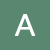adurden2012
Owner
7 items   1 followers   0 votes   5 views

# Fractions

addition, subtraction, division, multiplication of fractions and fractions with manipulative

151

## Intro to equivalent fractionsSal introduces equivalent fractions with pizza.

156

## Fraction Manipulatives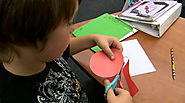Watch a Math lesson on fractions using manipulatives. Students use fractions strips and circle graphs along with the build it, draw it, write it method to gain a better understanding of fractions. Great lesson to get the students to lead their own learning.

157

## Renaming FractionsThe following section reviews the processes of renaming and simplifying fractions. These processes are invaluable when you add, subtract, multiply, or divide.

Love that they use the term "rename" fractions and not "reduce". Often when we use the word reduce fractions kids can get confused. However I dislike the way they use cross product and simplifying fractions. There are equally simple models that would make the transition to algebra easier.

158

## Fraction Number Line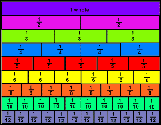Math explained in easy language, plus puzzles, games, quizzes, worksheets and a forum. For K-12 kids, teachers and parents.

Its a great resource to show students you don't "reduce" the fraction, you are simply renaming it. Also great to compare what fractions are larger. bigger digits doesn't imply bigger fractions i.e. 2/3 vs 6/10

159

## 1. Fractions as division (C)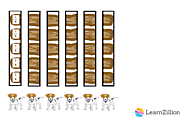helps students understand that 5 divided by 6 is 5/6. This example is given by 6 dogs equally sharing 5 treats. Great visual demonstration.

160

## Rule 10: Reducing Fractions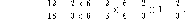Rule 10: Reducing Fractions using arithmetic to algebra methods.

161

## Grab One!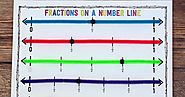Great list! I never thought of using dominoes during my fraction work! I can think of some fun and quick ways to use them as random fraction generators for a variety of games. Thanks! TaraThe Math Maniac